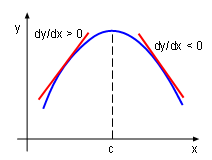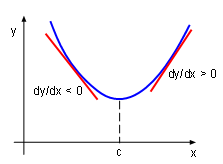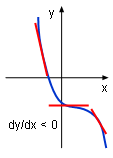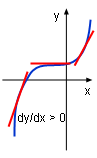Ch 4. The Mean Value Theorem Multimedia Engineering Math Maximum & Minimum Rolle'sTheorem Mean ValueTheorem MonotonicFunctions First DerivativeTest Concavity &Inflection SecondDerivative Test
 Chapter 1. Limits 2. Derivatives I 3. Derivatives II 4. Mean Value 5. Curve Sketching 6. Integrals 7. Inverse Functions 8. Integration Tech. 9. Integrate App. 10. Parametric Eqs. 11. Polar Coord. 12. Series Appendix Basic Math Units Search eBooks Dynamics Fluids Math Mechanics Statics Thermodynamics Author(s): Hengzhong Wen Chean Chin Ngo Meirong Huang Kurt Gramoll ©Kurt GramollMATHEMATICS - THEORY The First Derivative Test Recall that Fermat's Theorem states that if a function has a local extreme value at a point, then that point is a critical point of the function. However, a critical point does not guarantee an extreme value. Thus, a test is needed to determine whether a local extreme value is located at a critical point or not. It is called the first derivative test. It states:Local MaximumLocal MinimumNo Extreme Suppose x0, is a critical point of a continuous function. If the value of the function's derivative changes from positive to negative at x0, then the function has a local maximum at this point. If the value of the function's derivative changes from negative to positive at x0, then the function has a local minimum at this point. If the value of the function's derivative does not change sign at this point, then the function has no local extreme at this point. The concept of the first derivative test can be better understand by explaining the following example. Examining the case when the derivative of a function changes from positive to negative as shown on the left graphic. When x < c, the derivative of the function is positive, according to the Test for Monotonic Functions the function is increasing. That is f(c) > f(x) when x < c. When x > c, the derivative of the function is negative, according to the Test for Monotonic Functions the function is decreasing. That is f(c) > f(x) when x > c. Thus, f(c) is the local maximum according to the local maximum definition.No Extreme